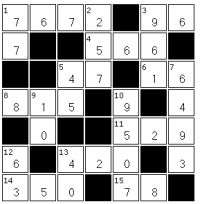# Maths Crossword PuzzlesThe CrossNumber puzzle is a little like a crossword, but instead of using words it uses numbers - hence the name of CrossNumber!

We have 100 Cool Crossnumber puzzles for you to get your teeth into here at Childrens Puzzles. If you can complete them all then you're a whizz at mental arithmetic and sums!

All you have to do is work out the answer to each of the sums and write the numbers in the grid. If you get stuck you can view the solution or check you got it right if you finish a puzzle. There are five types of clue:

• Number word clues: here the number is written in letters and you need to work out the correct number. So "five hundred and thirty four" becomes '534'
• Addition or sum clues: e.g. 50 + 2 is '52' so enter '52' in the grid
• Subtraction or minus clues: e.g. 50 - 2 is '48' so enter '48' in the grid
• Multiplication or times clues: e.g. 26 x 2 is '52' so enter '52' in the grid
• Division clues: e.g. 104 / 2 is '52' so enter '52' in the grid

Think you can handle the puzzles? Then Play a Sample Cross Number puzzle >>>